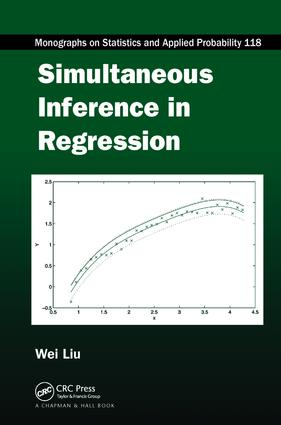# Simultaneous Inference in Regression

## 1st Edition

CRC Press

292 pages | 89 B/W Illus.

##### Purchasing Options:\$ = USD
Paperback: 9781138111684
pub: 2017-06-13
\$82.95
x
Hardback: 9781439828090
pub: 2010-10-19
Currently out of stock
\$220.00
x
eBook (VitalSource) : 9780429094354
pub: 2010-10-19
from \$39.98

FREE Standard Shipping!

### Description

Simultaneous confidence bands enable more intuitive and detailed inference of regression analysis than the standard inferential methods of parameter estimation and hypothesis testing. Simultaneous Inference in Regression provides a thorough overview of the construction methods and applications of simultaneous confidence bands for various inferential purposes. It supplies examples and MATLAB® programs that make it easy to apply the methods to your own data analysis. The MATLAB programs, along with color figures, are available for download on www.personal.soton.ac.uk/wl/mybook.html

Most of the book focuses on normal-error linear regression models. The author presents simultaneous confidence bands for a simple regression line, a multiple linear regression model, and polynomial regression models. He also uses simultaneous confidence bands to assess part of a multiple linear regression model with the zero function, to compare two regression models, and to evaluate more than two regression models. The final chapter demonstrates the use of simultaneous confidence bands in generalized linear regression models, such as logistic regression models.

This book shows how to employ simultaneous confidence bands to make useful inferences in regression analysis. The topics discussed can be extended to functions other than parametric regression functions, offering novel opportunities for research beyond linear regression models.

### Reviews

I like in particular this book since almost all results are given with proofs and that these proofs are easy to understand having some knowledge in linear models. It is especially helpful that the geometric ideas behind the proofs are worked out and presented with many illustrations. Together with the comprehensive review of the published literature and the presentation of some unsolved problems, this book is very valuable for researchers. It is also very recommendable for practitioners since the ideas of the concepts are worked out clearly and many examples and figures are presented where the confidence bands are applied to real data. MATLAB programs for all methods can be downloaded from the author’s website.

—Christine Müller, Biometrical Journal 53 (2011)

This book provides a comprehensive discussion of methods for determining simultaneous confidence bands in regression. … The book provides a valuable up-to-date review of work in this area.

—David J. Hand, International Statistical Review (2011), 79

… definitely fills a significant niche, providing practitioners with powerful inference tools for parametric regression and stimulating further research in this important area.

— Tatyana Krivobokova, Georg-August- Univer,citat Gottingen, Royal Statistics Society, February 2012

Introduction to Linear Regression Analysis

Linear regression models

Parameter estimation

Testing hypotheses

Confidence and prediction intervals

Confidence Bands for One Simple Regression Model

Preliminaries

Hyperbolic bands

Three-segment bands

Two-segment bands

Other confidence bands

Extensions and restrictions of a confidence band

Comparison of confidence bands

Confidence bands for percentiles and tolerance bands

Bayesian simultaneous credible bands

Confidence Bands for One Multiple Regression Model

Hyperbolic bands over the whole space

Hyperbolic bands over a rectangular region

Constant width bands over a rectangular region

Hyperbolic bands over an ellipsoidal region

Constant-width bands over an ellipsoidal region

Other confidence bands

Assessing Part of a Regression Model

Partial F test approach

Hyperbolic confidence bands

Assessing equivalence to the zero function

Comparison of Two Regression Models

Partial F test approach

Hyperbolic bands over the whole space

Confidence bands over a rectangular region

Confidence bands over an ellipsoidal region

Assessing the equivalence of two models

Comparison of More Than Two Regression Models

Partial F test approach

Hyperbolic confidence bands for all contrasts

Bands for finite contrasts over rectangular region

Bands for finite contrasts over ellipsoidal region

Equivalence of more than two models

Confidence Bands for Polynomial Regression

Confidence bands for one model

Confidence bands for part of a polynomial model

Comparison of two polynomial models

Comparison of more than two polynomial models

Confidence Bands for Logistic Regression

Introduction to logistic regression

Bands for one model

Bands for comparing two models

Bands for comparing more than two models

Appendix A: Approximation of the Percentile of a Random Variable

Appendix B: Computation of Projection π (t,P,Xr)

Appendix C: Computation of Projection π*(t,W,X2)

Appendix D: Principle of Intersection-Union Test

Appendix E: Computation of the K-Functions in Chapter 7

Bibliography

Index

### About the Author

Wei Liu is a professor of statistics at the University of Southampton, UK. Dr. Liu has published more than 80 papers in peer-reviewed journals, including Annals of Statistics, Journal of the American Statistical Association, Journal of the Royal Statistical Society, Biometrika, and Biometrics. His research encompasses multiple comparison, simultaneous inference, and sequential methods.

### Subject Categories

##### BISAC Subject Codes/Headings:
MAT029000
MATHEMATICS / Probability & Statistics / General